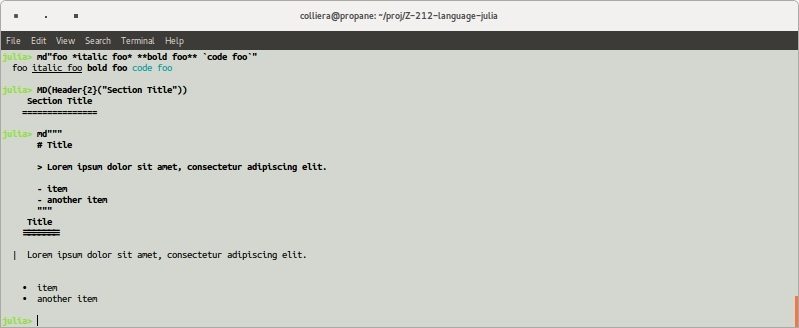Day 36: MarkdownMarkdown is a lightweight format specification language developed by John Gruber. Markdown can be converted to HTML, LaTeX or other document formats. You probably knew all that already. The syntax is pretty simple. Check out this useful cheatsheet.

In the latest stable version of Julia support for markdown is provided in the Base package.

julia> using Base.Markdown
julia> import Base.Markdown: MD, Paragraph, Header, Italic, Bold, LineBreak, plain, term, html,
Table, Code, LaTeX, writemime

Markdown is stored in objects of type Base.Markdown.MD. As you’ll see below, there are at least two ways to construct markdown objects: either directly from a string (using markdown syntax) or programmatically (using a selection of formatting functions).

julia> d1 = md"foo \*italic foo\* \*\*bold foo\*\* \code foo\";
julia> d2 = MD(Paragraph(["foo ", Italic("italic foo"), " ", Bold("bold foo"), " ",
Code("code foo")]));
julia> typeof(d1)
Base.Markdown.MD
julia> d1 == d2
true

You’ll find that Base.Markdown.MD objects are rendered with appropriate formatting in your console.Functions html() and latex() convert Base.Markdown.MD objects into other formats. Another way of rendering markdown elements is with writemime(), where the output is determined by specifying a MIME type.

julia> html(d1)
"<p>foo <em>italic foo</em> <strong>bold foo</strong> <code>code foo</code></p>\n"
julia> latex(d1)
"foo \\emph{italic foo} \\textbf{bold foo} \\texttt{code foo}\n"

Markdown has support for section headers, both ordered and unordered lists, tables, code fragments and block quotes.

julia> d3 = md"""# Chapter Title
## Section Title
### Subsection Title""";
julia> d3 |> html
"<h1>Chapter Title</h1>\n<h2>Section Title</h2>\n<h3>Subsection Title</h3>\n"
julia> latex(d4)
"\\subsection{Section Title}\n"

Most Julia packages come with a README.md markdown file which provides an overview of the package. The readme() function gives you direct access to these files' contents.

Quandl.jl
============
(Image: Build Status)
(Image: Coverage Status)
(Image: Quandl)

Documentation is provided by Read the Docs.

See the Quandl API Help Page for further details about the Quandl API. This package
closely follows the nomenclature used by that documentation.
We can also use parse_file() to treat the contents of a file as markdown.

This is rendered below as LaTeX.

\section{NaNMath}
Implementations of basic math functions which return \texttt{NaN} instead of throwing a
\texttt{DomainError}.
Example:
\begin{verbatim}
import NaNMath
NaNMath.log(-100) # NaN
NaNMath.pow(-1.5,2.3) # NaN
\end{verbatim}
In addition this package provides functions that aggregate one dimensional arrays and ignore
elements that are NaN. The following functions are implemented:
\begin{verbatim}
sum
maximum
minimum
mean
var
std
\end{verbatim}
Example:
\begin{verbatim}
using NaNMath; nm=NaNMath
nm.sum([1., 2., NaN]) # result: 3.0
\end{verbatim}
\href{https://travis-ci.org/mlubin/NaNMath.jl}{\begin{figure}
\centering
\includegraphics{https://travis-ci.org/mlubin/NaNMath.jl.svg?branch=master}
\caption{Build Status}
\end{figure}
}

And here it is as HTML.

NaNMath

Implementations of basic math functions which return NaN instead of throwing a DomainError.
Example:

import NaNMath
NaNMath.log(-100) # NaN
NaNMath.pow(-1.5,2.3) # NaN

In addition this package provides functions that aggregate one dimensional arrays and ignore elements that are NaN. The following functions are implemented:

sum
maximum
minimum
mean
var
std

Example:

using NaNMath; nm=NaNMath
nm.sum(([)[1., 2., NaN]) # result: 3.0

What particularly appeals to me about the markdown functionality in Julia is the potential for automated generation of documentation and reports. To see more details of my dalliance with Julia and markdown, visit github.